# Latent Dirichlet Allocation

$\newcommand{\EE}{\mathbb{E}} \newcommand{\wb}{\mathbf{w}} \newcommand{\zb}{\mathbf{z}} \newcommand{\Poisson}{\text{Poisson}} \newcommand{\Dir}{\text{Dir}} \newcommand{\Multinomial}{\text{Multinomial}}$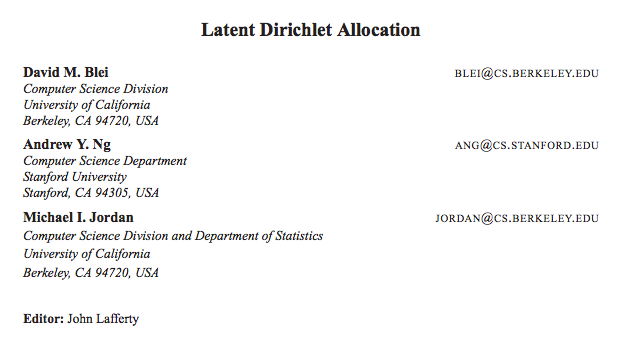* Picture are reproduced from original paper

• A word is the basic unit of discrete data, defined to be an item from a vocabulary indexed by $\{1,…,V\}$.

• A document is a sequence of N words denoted by $\wb = (w_1,w_2,…,w_N)$, where $w_n$ is the nth word in the sequence.

• A corpus is a collection of M documents denoted by $D = \{w_1,w_2,…,w_M\}$.

We wish to find a probabilistic model of a corpus that not only assigns high probability to members of the corpus, but also assigns high probability to other “similar” documents.

## Latent Dirichlet Allocation

1. Choose $N \sim \Poisson(\zeta)$.
2. Choose $\theta \sim \Dir(\alpha)$.
3. For each of the $N$ words $w_n$:

(a) Choose a topic $z_n \sim \Multinomial(\theta)$.

(b) Choose a word $w_n$ from $p(w_n \vert z_n,\beta)$, a multinomial probability conditioned on the topic $z_n$.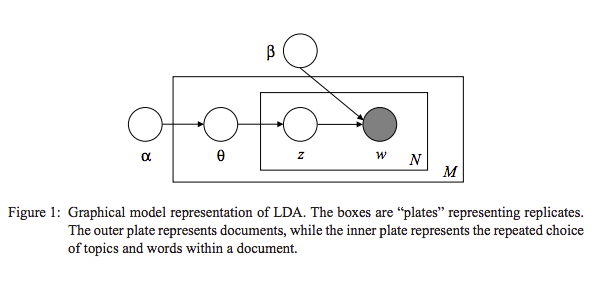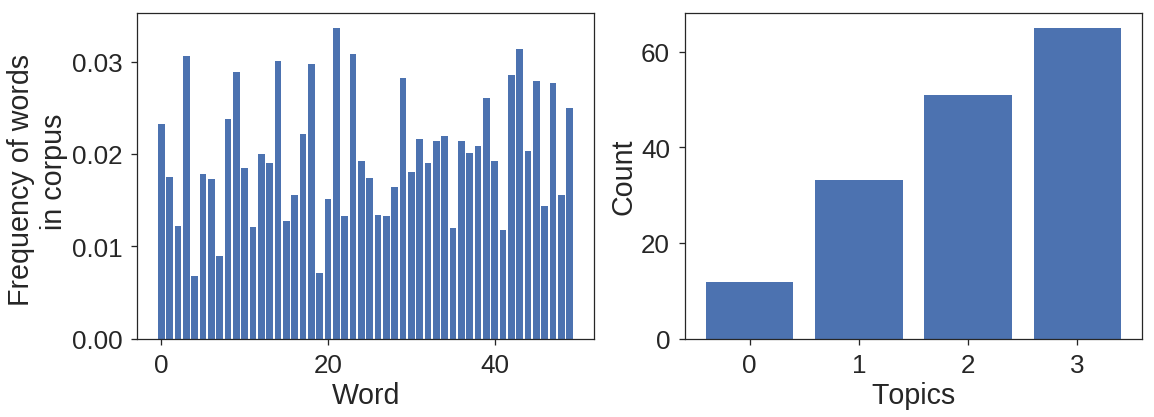### Likelihood function

Given parameters $\alpha$ and $\beta$, the joint distribution of a topic mixture $\mathbf{\theta}$, a set of $N$ topics $\mathbf{z}$, and a set of $N$ words $\mathbf{w}$ is given by:

\begin{align} p(\mathbf{\theta},\mathbf{z},\mathbf{w}\vert \alpha,\beta)=p(\mathbf{\theta}\vert \alpha)\prod_{n=1}^{N}p(z_n\vert \mathbf{\theta})p(w_n\vert z_n,\beta) \end{align}

We only observe $\wb$ while $\theta$ and $\zb$ are not observed. Integrating over $\mathbf{\theta}$ and summing over $z$, we get marginal probabilities of a document:

\begin{align} p(\mathbf{w}\vert \alpha,\beta)=\int p(\mathbf{\theta}\vert \alpha)\big(\prod_{n=1}^{N}\sum_{z_{dn}}p(z_n\vert \mathbf{\theta})p(w_n\vert z_n,\beta)\big)d\theta \end{align}

Finally the likelihood of observing a corpus is:

\begin{align} p(D\vert \alpha,\beta)=\prod_{d=1}^{M}\int p(\theta_d \vert \alpha)\big(\prod_{n=1}^{N}\sum_{z_{dn}}p(z_n \vert \theta_d)p(w_n \vert z_n,\beta)\big)d\theta_d \end{align}

## Inference

We take a Bayesian perspective, and are interested in posterior distribtion after observing data.

for a given document we have:

\begin{align} p(\mathbf{\theta},\mathbf{z}\vert \mathbf{w},\alpha,\beta) = \frac{p(\mathbf{\theta},\mathbf{z},\mathbf{w}|\alpha,\beta)}{p(\mathbf{w}\vert \alpha,\beta)} \end{align}

Normalization constant in this distribution is intractable, therefore, we try other methods such as Variational Inference, Sampling or Approxiamtion.

### Variational Inference

The basic idea of convexity-based variational inference is to make use of Jensen’s inequality to obtain an adjustable lower bound on the log likelihood (Jordan et al., 1999). Essentially, one considers a family of lower bounds, indexed by a set of variational parameters. The variational parameters are chosen by an optimization procedure that attempts to find the tightest possible lower bound.

Take a prticular family of variational distributions: \begin{align} q(\mathbf{\theta},\mathbf{z}|\gamma,\phi) = q(\theta|\gamma)\prod_{n=1}^{N} q(z_n|\mathbf{\phi}_n) \end{align}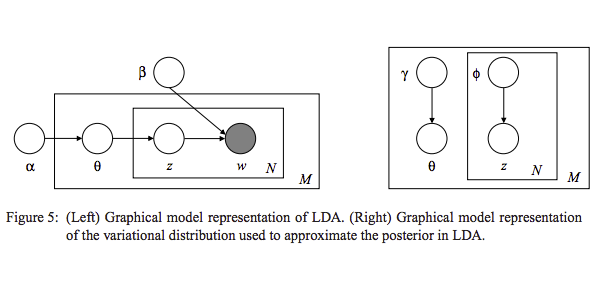Set up an optimization problem that determines the values of the variational parameters $\gamma$ and $\phi$:

\begin{align} (\gamma^{*},\phi^{*}) = \text{argmin}_{(\gamma,\phi)} \text{D}\big[q(\mathbf{\theta},\mathbf{z}|\gamma,\phi) ||p(\mathbf{\theta},\mathbf{z}|\mathbf{w},\alpha,\beta)\big]. \end{align}

Which results in following pair of update rules:

\begin{align} \phi_{ni} \propto \beta_{iv} \exp{(\Psi(\gamma_i)-\Psi(\sum_{j=1}^{k}\gamma_i))}. \end{align}

\begin{align} \gamma_{i} = \alpha_i+\sum_{n=1}^{N} \phi_{ni} \end{align}

#### Derivation

\begin{align} p(\mathbf{\theta},\mathbf{z},\mathbf{w}|\alpha,\beta)=p(\mathbf{\theta}|\alpha)\prod_{n=1}^{N}p(z_n|\mathbf{\theta})p(w_n|z_n,\beta) \end{align} \begin{align} \log{p(\mathbf{w}|\alpha,\beta}) =~& \log{\int \sum_{\mathbf{z}} p(\theta,\mathbf{z},\mathbf{w}|\alpha,\beta)}d\theta \nonumber\\
=~&\log{\int \sum_{\mathbf{z}}\frac{ p(\theta,\mathbf{z},\mathbf{w}|\alpha,\beta)q(\theta,\mathbf{z})}{q(\theta,\mathbf{z})}}d\theta\nonumber\\
\geq~&\int \sum_{\mathbf{z}} q(\theta,\mathbf{z})\log{p(\theta,\mathbf{z},\mathbf{w}|\alpha,\beta)}-q(\theta,\mathbf{z})\log{q(\theta,\mathbf{z}})d\theta\tag*{Jensen’s inequality}\\
=~& \EE_q[{\log{p(\theta,\zb,\wb|\alpha,\beta})}]-\EE_q[\log{q(\theta,\zb)}] = L (\gamma,\phi;\alpha,\beta) \end{align}

\begin{align} L(\gamma,\phi;\alpha,\beta) = \EE_q[\log{p(\theta|\alpha)}]+ \EE_q[\log{p(\zb|\theta)}]+\EE_q[\log{p(\wb|\zb,\beta)}]- \EE_q[\log{q(\theta)}] - \EE_q[\log{q(\zb)}] \end{align}

\begin{align} L(\gamma,\phi;\alpha,\beta) &~= \log{\Gamma(\sum_{j=1}^k \alpha_j)} - \sum_{i=1}^k \log{\Gamma(\alpha_i)} + \sum_{i=1}^k (\alpha_i - 1)\big(\Psi(\gamma_i)-\Psi(\sum_{j=1}^k \gamma_j)\big)\\
&~+ \sum_{n=1}^N \sum_{i=1}^k \phi_{ni}\big(\Psi(\gamma_i) - \Psi(\sum_{j=1}^k \gamma_j)\big)\\
&~+ \sum_{n=1}^N \sum_{i=1}^k \phi_{ni}w_n^j \log{\beta_{ij}}\\
&~- \log{\Gamma(\sum_{j=1}^k \gamma_j)} + \sum_{i=1}^k \log{\Gamma(\gamma_i)} - \sum_{i=1}^k (\gamma_i - 1)(\Psi(\gamma_i)-\Psi(\sum_{j=1}^k \gamma_j))\\
&~- \sum_{n=1}^N \sum_{i=1}^k \phi_{ni}\log{\phi_{ni}} \end{align}

Maximizing the above equation wrt to each parameter:

\begin{align} L_{[\phi_{ni}]} =~& \phi_{ni}(\Psi(\gamma_i)-\Psi(\sum_{j=1}^{k}\gamma_i) + \phi_{ni}\log{\beta_{iv}} - \phi_{ni}\log(\phi_{ni}) + \lambda_n(\sum_{j=1}^{k} \phi_{nj} -1)\\
\frac{\partial L_{[\phi_{ni}]}}{\partial \phi_{ni}} =~& \Psi(\gamma_i)-\Psi(\sum_{j=1}^{k}\gamma_i) + \log{\beta_{iv}} - \log(\phi_{ni}) -1 + \lambda\\
\phi_{ni} \propto~& \beta_{iv} \exp{(\Psi(\gamma_i)-\Psi(\sum_{j=1}^{k}\gamma_i))}. \end{align}

\begin{align} L_{[\gamma_{i}]} =~& \sum_{i=1}^{k}(\alpha_i - 1)(\Psi(\gamma_i)-\Psi(\sum_{j=1}^{k}\gamma_j)) + \sum_{n=1}^{N} \phi_{ni}(\Psi(\gamma_i) - \Psi(\sum_{j=1}^{k}\gamma_j))\\
% &-\log{\Gamma(\sum_{j=1}^{k}\gamma_j)}+\log{\Gamma(\gamma_i)-\sum_{i=1}^{k}(\gamma_i-1)(\Psi(\gamma_i)-\Psi(\sum_{j=1}^{k}\gamma_j))}\\
%% =~& \sum_{i=1}^{k}(\Psi(\gamma_i)-\Psi(\sum_{j=1}^{k}\gamma_j)(\alpha_i + \sum_{n=1}^{N}\phi_{ni}-\gamma_{i})-\log{\Gamma(\sum_{j=1}^{k}\gamma_j)}+\log{\Gamma(\gamma_i)}\\
% \frac{\partial L_{[\gamma_{i}]}}{\partial\gamma_{i}} =~& \Psi’(\gamma_i)(\alpha_i + \sum_{n=1}^{N}\phi_{ni}-\gamma_{i})-\Psi’(\sum_{j=1}^{k}\gamma_j)\sum_{j=1}^{k}(\alpha_j + \sum_{n=1}^{N}\phi_{nj}-\gamma_{j})\\
\gamma_{i} =~& \alpha_i+\sum_{n=1}^{N} \phi_{ni} \end{align}

## Experiments

diff gamma 133.635209 diff phi 0.014895 L -23.776968
diff gamma 25.256380 diff phi 0.000735 L -23.141265
diff gamma 22.212411 diff phi 0.000636 L -22.511695
diff gamma 18.884462 diff phi 0.000534 L -21.896922
diff gamma 15.560183 diff phi 0.000436 L -21.305556
diff gamma 12.495897 diff phi 0.000349 L -20.745106
diff gamma 9.842222 diff phi 0.000274 L -20.221272
diff gamma 7.646850 diff phi 0.000213 L -19.737676
diff gamma 5.888301 diff phi 0.000164 L -19.295954
diff gamma 4.510381 diff phi 0.000126 L -18.896069
diff gamma 3.446265 diff phi 0.000097 L -18.536718
diff gamma 2.631902 diff phi 0.000074 L -18.215718
diff gamma 2.011879 diff phi 0.000057 L -17.930349
diff gamma 1.540939 diff phi 0.000044 L -17.677624
diff gamma 1.183386 diff phi 0.000034 L -17.454480
diff gamma 0.911664 diff phi 0.000026 L -17.257917
diff gamma 0.704776 diff phi 0.000020 L -17.085083
diff gamma 0.546850 diff phi 0.000016 L -16.933323
diff gamma 0.425937 diff phi 0.000012 L -16.800206
diff gamma 0.333056 diff phi 0.000010 L -16.683532
diff gamma 0.261459 diff phi 0.000008 L -16.581325
diff gamma 0.206069 diff phi 0.000006 L -16.491823
diff gamma 0.163057 diff phi 0.000005 L -16.413463
diff gamma 0.129534 diff phi 0.000004 L -16.344865
diff gamma 0.103307 diff phi 0.000003 L -16.284812
diff gamma 0.082711 diff phi 0.000002 L -16.232234
diff gamma 0.066476 diff phi 0.000002 L -16.186193
diff gamma 0.053631 diff phi 0.000002 L -16.145866
diff gamma 0.043431 diff phi 0.000001 L -16.110535
diff gamma 0.035300 diff phi 0.000001 L -16.079568
diff gamma 0.028795 diff phi 0.000001 L -16.052417
diff gamma 0.023572 diff phi 0.000001 L -16.028601
diff gamma 0.019363 diff phi 0.000001 L -16.007698
diff gamma 0.015959 diff phi 0.000000 L -15.989344
diff gamma 0.013197 diff phi 0.000000 L -15.973218
diff gamma 0.010948 diff phi 0.000000 L -15.959041
diff gamma 0.009111 diff phi 0.000000 L -15.946569
diff gamma 0.007605 diff phi 0.000000 L -15.935589
diff gamma 0.006366 diff phi 0.000000 L -15.925916
diff gamma 0.005344 diff phi 0.000000 L -15.917387
diff gamma 0.004498 diff phi 0.000000 L -15.909861
diff gamma 0.003796 diff phi 0.000000 L -15.903213
diff gamma 0.003211 diff phi 0.000000 L -15.897338
diff gamma 0.002723 diff phi 0.000000 L -15.892138
diff gamma 0.002315 diff phi 0.000000 L -15.887534
diff gamma 0.001972 diff phi 0.000000 L -15.883451
diff gamma 0.001683 diff phi 0.000000 L -15.879829
diff gamma 0.001440 diff phi 0.000000 L -15.876610
diff gamma 0.001234 diff phi 0.000000 L -15.873747
diff gamma 0.001059 diff phi 0.000000 L -15.871198
iter 50
diff gamma 40.601717 diff phi 0.232107
diff beta 0.000000

<matplotlib.text.Text at 0x7fde04dfa5c0>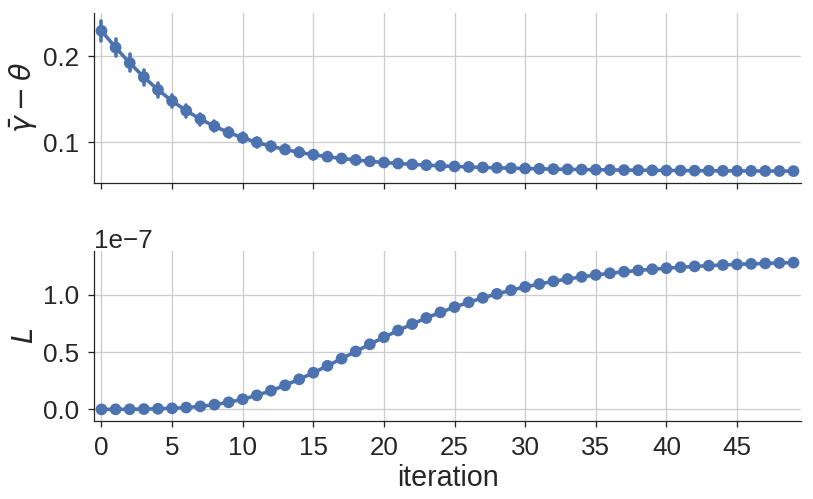Tags:

Categories:

Updated: